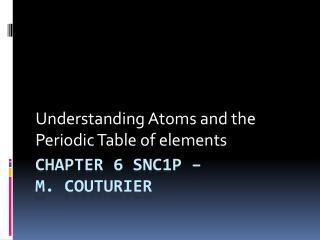DownloadDownload PresentationChapter 6 SNC1P – m. Couturier

# Chapter 6 SNC1P – m. Couturier

Télécharger la présentation## Chapter 6 SNC1P – m. Couturier

- - - - - - - - - - - - - - - - - - - - - - - - - - - E N D - - - - - - - - - - - - - - - - - - - - - - - - - - -
##### Presentation Transcript

1. Understanding Atoms and the Periodic Table of elements Chapter 6 SNC1P – m. Couturier

2. The atom • Atoms are made up of three sub-atomic particles: protons, neutrons and electrons. • Protons are positively charged particles which reside in the nucleus of an atom. It is the proton that defines the element. • Neutrons are neutral particles which also reside in the nucleus of an atom. The neutron defines the isotope. • Electrons are negatively charge particles which orbit the nucleus of the atom. The electron defines the ion or the atoms charge.

3. The atom

4. The atomic number • Recall that the number of protons defines the element. This number is known as the atomic number, which is located on the top left of the element box within the PTE. • Knowing the atomic number means you know the element. Ex. Lithium has an atomic number of 3 and therefore has 3 protons. • Can you identify which element has 35 protons?

5. Iodine’s Atomic Number is 53

6. The atomic mass • The atomic mass is in essence the mass of a single atom. The mass is combined mass of the protons, neutrons and electrons. The atomic mass of Iodine is 126.90 μ. It is found below the element symbol. • What is the atomic mass of Calcium?

7. The mass number • The mass number is the summation of the protons and neutrons within the atom’s nucleus. The mass number is essential when trying to determine the number of neutrons (assuming you are given the atomic number) and the isotope you are dealing with. Example: Carbon-12 and Carbon-14 are isotopes of carbon. The number 12 an 14 are their mass numbers respectively.

8. The mass number • In the case of Carbon-12, we can deduce that: • Carbon-12’s mass number is 12 (given). • Carbon has 6 protons (implied). • Carbon-12 has 6 neutrons (12-6=6) • In the case of Carbon-14, we can deduce that : • Carbon-14’s mass number is 14 (given) • Carbon has 6 protons (implied) • Carbon-14 has 8 neutrons (14-6=8)

9. Mass Number • So in essence, we can find the number of neutrons if we are given the mass number because: • Mass Number = Atomic Number + Neutrons • or • Neutrons = Mass Number – Atomic Number • Recall that the Atomic Number is the number of protons

10. Charge Number • The charge number is the electrical difference between protons and electrons. Since protons are positive and electrons are negative, each particle cancels out the next. • Charge Number = #protons + #electons • Example: Na, or sodium always has 11 protons. If the atom has 11 electrons, then the charge number is 0, hence the atom is neutral. Since • Charge Number  11 + (-11) = 0

11. Charge Number • More examples: • In the unlikely event that Na would have 12 electrons, then the charge number would be -1 which means the atom is negatively charged. Recall that the protons never change. • Charge Number = 11 + (-12) = -1. • The more likely outcome is that Na would have 10 electrons, hence the charge number would be +1 which means the atom is positively charged. • Charge Number = 11 + (-10) = +1.

12. Standard Atomic Notation • To maximize the amount of information given, we present the data in the form of the standard atomic notation. • The element symbol is placed in the centre • The atomic number is given on the bottom left • The mass number is given on the top left • The charge number is given on the top right (if no number appears then the net charge is 0).

13. Standard Atomic Notation

14. Standard Atomic Notation • From the previous diagram we can state that: • Sodium is the element (Na) • Sodium has 11 protons (atomic number) • Sodium-23 (isotope) has 12 neutrons because (23- 11=12) • There is a +1 charge, hence there are only 10 electrons because (+1 = +11 – 10).

15. Standard Atomic Notation